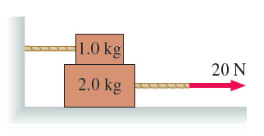# Problem: The 10 kg block in the figure is tied to the wall with a rope. It sits on top of the 2.0 kg block. The lower block is pulled to the right with a tension force of 20 N. The coefficient of kinetic friction at both the lower and upper surfaces of the 2.0 kg block is μk = 0.38. Part A. What is the tension in the rope holding the 1.0 kg block to the wall? Part B. What is the acceleration of the 2.0 kg block?

###### FREE Expert Solution

Part A

Let:

• The friction between the two blocks be Ff1
• The friction between the large block and the ground be Ff2
• The small mass be m1
• The large mass be m2

Taking the sum of forces in the y-direction:

T - Ff1 = 0

T1 = Ff1

But Ff1 = N1μk

98% (297 ratings)###### Problem Details

The 10 kg block in the figure is tied to the wall with a rope. It sits on top of the 2.0 kg block. The lower block is pulled to the right with a tension force of 20 N. The coefficient of kinetic friction at both the lower and upper surfaces of the 2.0 kg block is μk = 0.38.Part A. What is the tension in the rope holding the 1.0 kg block to the wall?

Part B. What is the acceleration of the 2.0 kg block?